# MID Function Examples – Excel, VBA, & Google Sheets

This tutorial demonstrates how to use the Excel MID Function in Excel to extract text from a cell.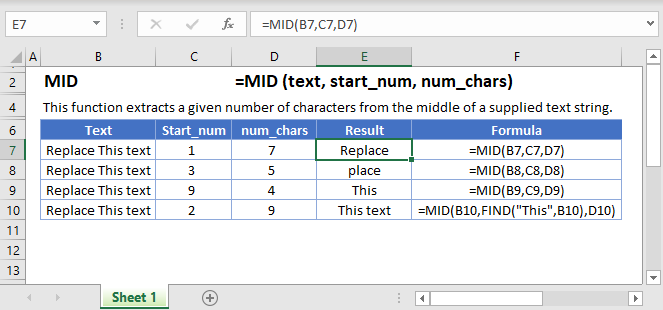## MID Function Overview

The MID Function Returns text from the middle of a cell, defined by a start number and the number of characters.

To use the MID Excel Worksheet Function, select a cell and type: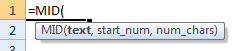(Notice how the formula inputs appear)

### MID function Syntax and inputs:

`= MID(text,start_num,num_chars)`

text – A string of text.

start_num – The character number from which to start your selection.

num_chars – The number of characters after the start_num to return.

## How to use the MID Function in Excel:

The MID function extracts a specific number of characters you specify from anywhere you want.

`=MID(B3,C3,D3)`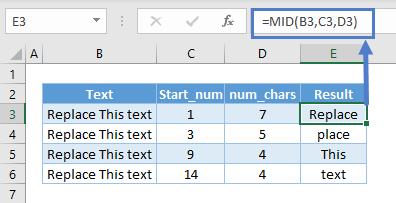It can start from any number seen in column C and any number of characters in column D.

### MID to Grab String between Different Delimiter

If the string you want is between two different delimiters, this is a pretty straightforward one. For instance, we might want the string between dash (“-”) and slash (“/”).

`=MID(B3,FIND("-",B3)+1,FIND("/",B3)-FIND("-",B3)-1)`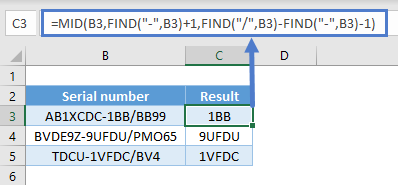In this case here, we can use FIND to check which character position the dash starts and add 1 to start extracting from there.

Use FIND again to check which character position the slash starts and minus the position of the dash and 1 to obtain the length of the string we want.

You can also use SEARCH. The difference between FIND and SEARCH is that the former is case-sensitive. Finding a symbol such as space wouldn’t matter.

`=MID(B3,FIND("-",B3)+1,SEARCH("/",B3)-SEARCH("-",B3)-1)`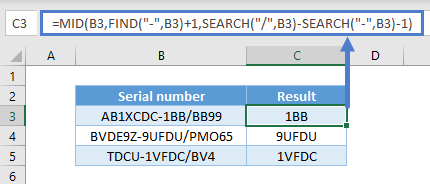### MID to Grab String between the Same Delimiter

If the string has the same delimiter, it is a little tougher than the one above because FIND grabs the first occurrence. For instance, we might want the string between the first and second spaces. But you can indicate a starting position in FIND as well.

`=MID(B3,FIND(" ",B3)+1,FIND(" ",B3,FIND(" ",B3)+1)-FIND(" ",B3)-1)`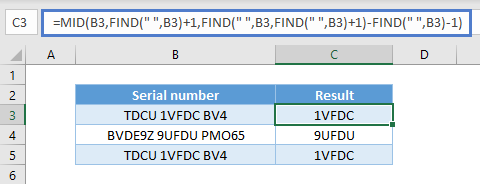It uses FIND just like the above example to check which character position the space starts and add 1 to start extracting from there.

Use FIND again to check which character position the second space starts by starting to find 1 character after the position of the first space.

And lastly minus the position of the first space and 1 to obtain the length of the string we want.

You can also use SEARCH as well.

`=MID(B3,FIND(" ",B3)+1,SEARCH(" ",B3,FIND(" ",B3)+1)-SEARCH(" ",B3)-1)`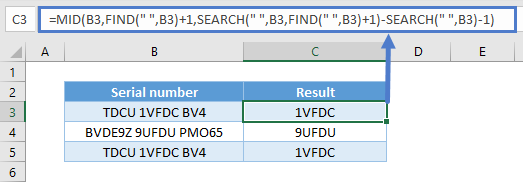### MID with Number/Dates

Do note that MID is a text function. Upon using MID, the result is a text. For instance, you won’t be able to sum up these numbers in cell E3 after using MID.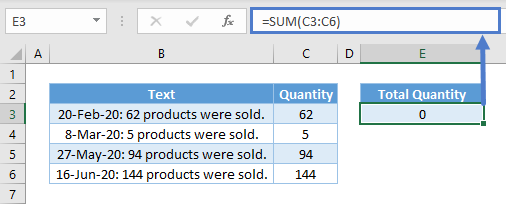The same thing happens for dates as they are recognized as serial numbers and not text. You may not need to sum dates, but it doesn’t work well in filters and PivotTables.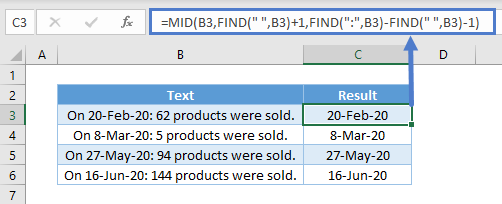To overcome the issues above, you can use VALUE to convert from text to values.

`=VALUE(MID(B3,FIND(":",B3)+2,FIND(" ",B3,FIND(" ",B3)+1)-FIND(":",B3)-2))`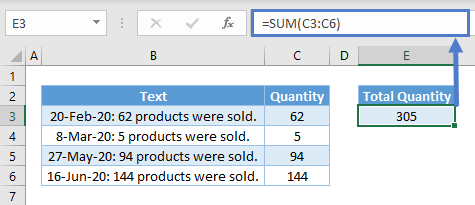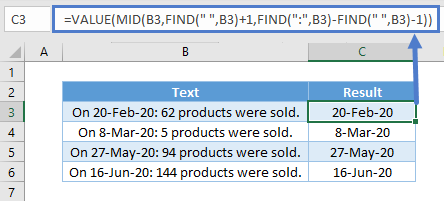### MID to Split by Common Delimiter

You might want to split all the words in different rows. Hence:

`=TRIM(MID(SUBSTITUTE(B\$3," ",REPT(" ",LEN(B\$3))),(ROWS(B3:B\$3)-1)*LEN(B\$3)+1,LEN(B\$3)))`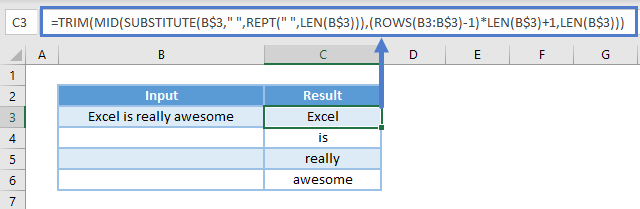What is does is use SUBSTITUTE to substitute all the spaces with an influx of repeated spaces using REPT. It results in the text looking like this:
“Excel                       is                       really                       awesome”

The influx of repeated is carefully repeated with a total length of the original text by using LEN. That means the position number to start extracting from is a multiple of the total length. For the first word, extract from position 1. For the second, it will be total length + 1. For the third, total length x 2 + 1. Etc. To get the nth word, use ROWS.

Lastly, the number of characters is always using the total length of the original text.

### Find Nth word in the String

We could combine TRIM, LEN, SUBSTITUTE, REPT  with MID as follows to get last word of the string.

`=TRIM(MID(SUBSTITUTE(A\$2," ",REPT(" ",LEN(A\$2))), (B2-1)*LEN(A\$2)+1, LEN(A\$2)))`

Which would give us the following results: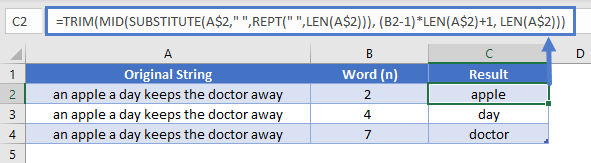## Reverse a text string

You can reverse a text string by combining MID function with TEXTJOINT as follow.

`=TEXTJOIN("",1,MID(A2,{10,9,8,7,6,5,4,3,2,1},1))`

Which would give us the following results: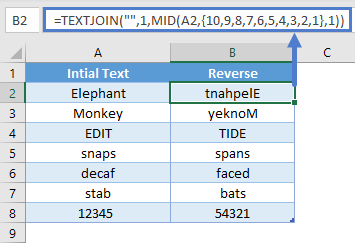The MID Function works exactly the same in Google Sheets as in Excel: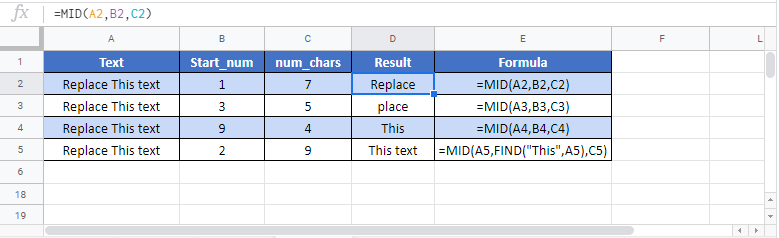`application.worksheetfunction.mid(text,start_num,num_chars)`